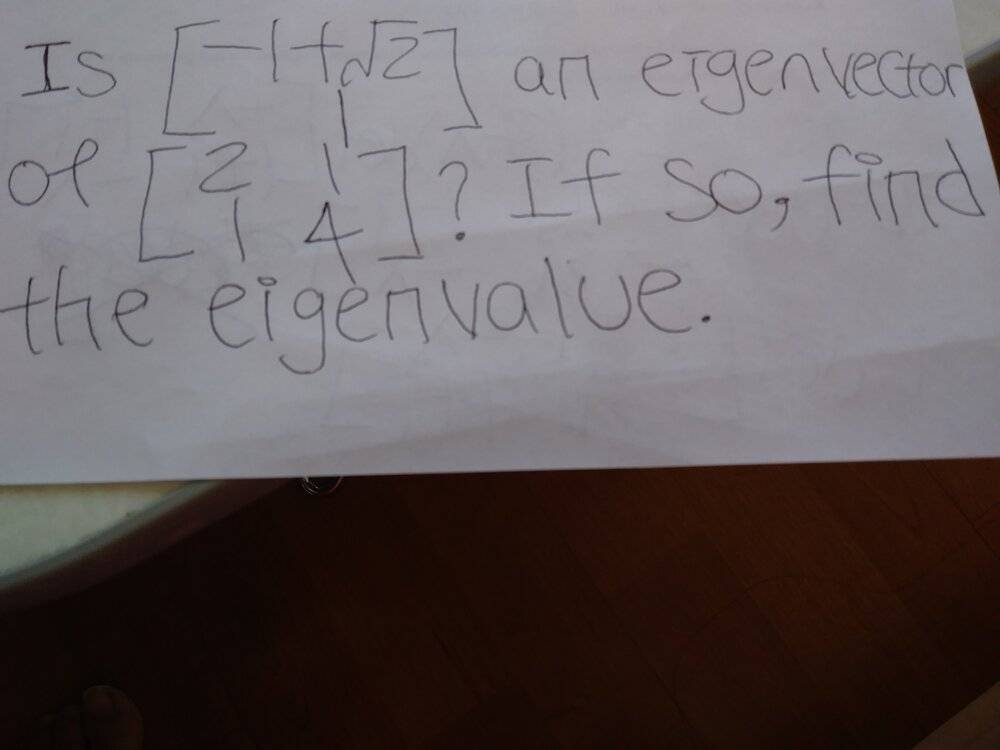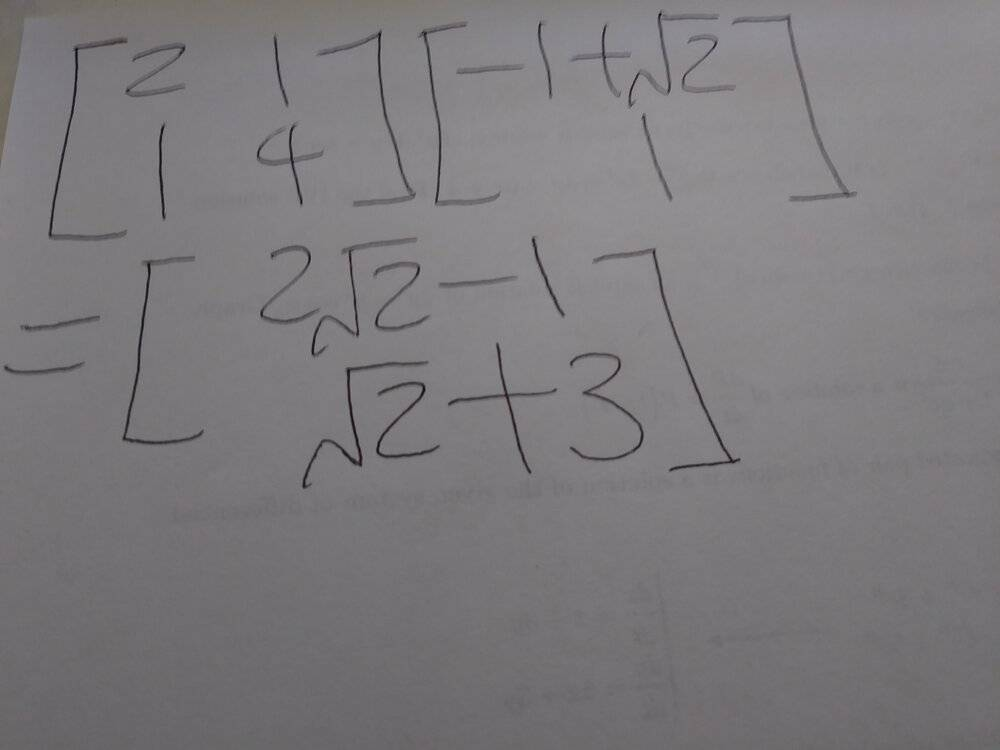# Can anyone please check my work and answer for this math problem?

• Math100

#### Math100

Homework Statement
Is [-1+sqrt(2), 1] an eigenvector of [2, 1; 1, 4]? If so, find the eigenvalue.
Relevant Equations
None.
Can anyone please confirm and check my work with answer because I'm not 100% sure if my work with answer is 100% correct. The question is asking if the given vector is an eigenvector and I've answered no. What's the correct answer for this problem? It's Yes or No?I think you need to write a little bit larger. Got any A3 nearby?

Sorry, I don't understand. What do you mean about A3 nearby?

Nevermind, it was just a bad attempt at humour. As for your question, is the vector ##
\begin{pmatrix}
2\sqrt{2} - 1\\
\sqrt{2} +3
\end{pmatrix}## a scalar multiple of the vector ##
\begin{pmatrix}
\sqrt{2} - 1\\
1
\end{pmatrix}##?

I think the answer is Yes, because (√2-1)(√2+3)=2√2-1. Therefore, it's scalar multiple. Am I right?

Right. And and if the result is a scalar multiple of the first vector, as in ##Av = \lambda v##, then ##v## is an eigenvector. Does that answer your question?•Math100
Yes. Thank you so much for the help.

•etotheipi